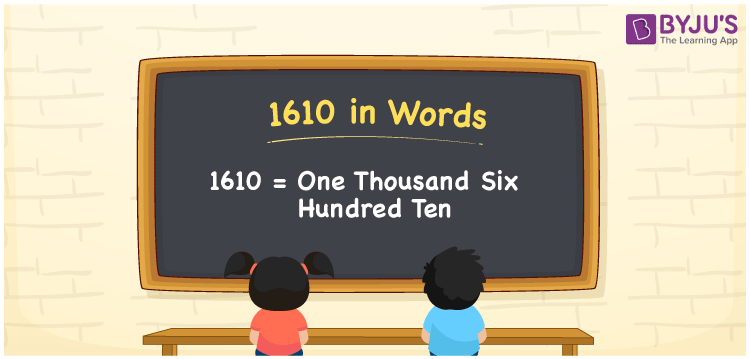# 1610 in Words

1610 in words can be written as One Thousand Six Hundred Ten. Students will be able to learn the conversion of 1610 in words which will help them understand the applications of numbers in our daily lives. If you buy a watch for Rs. 1610, then you can say that “I have bought a watch for One Thousand Six Hundred Ten Rupees”. The  number 1610 can be written in words using the English alphabet. The numbers in words can be grasped easily by the students using the resources given at BYJU’S. 1610 in English can be read as “One Thousand Six Hundred Ten”.

 1610 in words One Thousand Six Hundred Ten One Thousand Six Hundred Ten in Numbers 1610

## 1610 in English Words## How to Write 1610 in Words?

Students will learn about the conversion of 1610 into words from place value charts. The number 1610 has four digits. For 1610, the place value chart is given in a table form to help students understand it effectively.

 Thousands Hundreds Tens Ones 1 6 1 0

1610 in expanded form is explained in brief here:

1 × Thousand + 6 × Hundred + 1 × Ten + 0 × One

= 1 × 1000  + 6 × 100 + 1 × 10 + 0 × 1

= 1000 + 600 + 10

= 1610

= One Thousand Six Hundred Ten

Therefore, 1610 in words is written as One Thousand Six Hundred Ten.

1610 is a natural number that precedes 1611 and succeeds 1609.

1610 in words – One Thousand Six Hundred Ten

Is 1610 an odd number? – No

Is 1610 an even number? – Yes

Is 1610 a perfect square number? – Yes

Is 1610 a perfect cube number? – No

Is 1610 a prime number? – No

Is 1610 a composite number? – Yes

## Frequently Asked Questions on 1610 in Words

Q1

### How do you write 1610 in words?

1610 can be written as “One Thousand Six Hundred Ten” in words.
Q2

### Is 1610 an even number?

1610 is an even number because it is divisible by 2.
1610/2 = 805
Q3

### How can One Thousand Six Hundred Ten be written in numbers?

One Thousand Six Hundred Ten can be written in numbers as 1610.